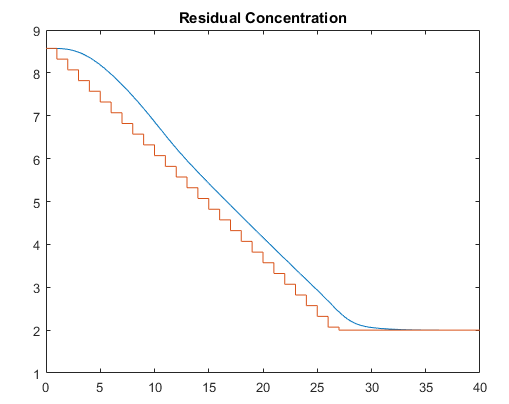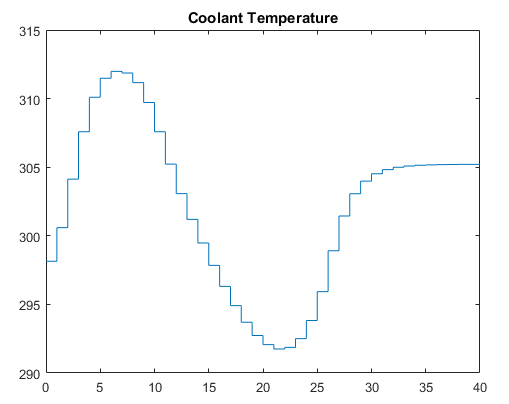## Simulate Linear MPC Controller with Nonlinear Plant using Successive Linearizations

You can use `sim` to simulate a closed-loop system consisting of a linear plant model and an MPC controller.

If your plant is a nonlinear Simulink® model, you can linearize the plant (see Linearization Using Model Linearizer in Simulink Control Design) and design a controller for the linear model (see Design MPC Controller in Simulink). To simulate the system, specify the controller in the MPC block parameter MPC Controller field and run the closed-loop Simulink model.

Alternatively, your nonlinear model might be a MEX-file, or you might want to include features unavailable in the MPC block, such as a custom state estimator. The `mpcmove` function is the Model Predictive Control Toolbox™ computational engine, and you can use it in such cases. The disadvantage is that you must duplicate the infrastructure that the `sim` function and the MPC block provide automatically.

### Nonlinear CSTR Application

The `CSTR` model described in Linearize Simulink Models is a strongly nonlinear system. As shown in Design MPC Controller in Simulink, a controller can regulate this plant, but degrades (and might even become unstable) if the operating point changes significantly.

The objective of this example is to redefine the predictive controller at the beginning of each control interval so that its predictive model, though linear, represents the latest plant conditions as accurately as possible. This will be done by linearizing the nonlinear model repeatedly, allowing the controller to adapt as plant conditions change. For more details on this approach, see  and .

### Example Code for Successive Linearization

In the following code, the simulation begins at the nominal operating point of the `CSTR` model (concentration = 8.57) and moves to a lower point (concentration = 2) where the reaction rate is much higher. The required code is as follows:

```[sys, xp] = CSTR_INOUT([],[],[],'sizes'); up = [10 298.15 298.15]; u = up(3); tsave = []; usave = []; ysave = []; rsave = []; Ts = 1; t = 0; while t < 40 yp = xp; % Linearize the plant model at the current conditions [a,b,c,d] = linmod('CSTR_INOUT',xp,up); Plant = ss(a,b,c,d); Plant.InputGroup.ManipulatedVariables = 3; Plant.InputGroup.UnmeasuredDisturbances = [1 2]; Model.Plant = Plant; % Set nominal conditions to the latest values Model.Nominal.U = [0 0 u]; Model.Nominal.X = xp; Model.Nominal.Y = yp; dt = 0.001; simOptions.StartTime = num2str(t); simOptions.StopTime = num2str(t+dt); simOptions.LoadInitialState = 'on'; simOptions.InitialState = 'xp'; simOptions.SaveTime = 'on'; simOptions.SaveState = 'on'; simOptions.LoadExternalInput = 'on'; simOptions.ExternalInput = '[t up; t+dt up]'; simOut = sim('CSTR_INOUT',simOptions); T = simOut.get('tout'); XP = simOut.get('xout'); YP = simOut.get('yout'); Model.Nominal.DX = (1/dt)*(XP(end,:)' - xp(:)); % Define MPC controller for the latest model MPCobj = mpc(Model, Ts); MPCobj.W.Output = [0 1]; % Ramp the setpoint r = max([8.57 - 0.25*t, 2]); % Compute the control action if t <= 0 xd = [0; 0]; x = mpcstate(MPCobj,xp,xd,[],u); end u = mpcmove(MPCobj,x,yp,[0 r],[]); % Simulate the plant for one control interval up(3) = u; simOptions.StartTime = num2str(t); simOptions.StopTime = num2str(t+Ts); simOptions.InitialState = 'xp'; simOptions.ExternalInput = '[t up; t+Ts up]'; simOut = sim('CSTR_INOUT',simOptions); T = simOut.get('tout'); XP = simOut.get('xout'); YP = simOut.get('yout'); % Save results for plotting tsave = [tsave; T]; ysave = [ysave; YP]; usave = [usave; up(ones(length(T),1),:)]; rsave = [rsave; r(ones(length(T),1),:)]; xp = XP(end,:)'; t = t + Ts; end figure(1) plot(tsave,[ysave(:,2) rsave]) title('Residual Concentration') figure(2) plot(tsave,usave(:,3)) title('Coolant Temperature') ```

### CSTR Results and Discussion

The plotted results appear below. Note the following points:

• The setpoint is being ramped from the initial concentration to the desired final value (see the step-wise changes in the reactor concentration plot below). The reactor concentration tracks this ramp smoothly with some delay (see the smooth curve), and settles at the final state with negligible overshoot. The controller works equally well (and achieves the final concentration more rapidly) for a step-wise setpoint change, but it makes unrealistically rapid changes in coolant temperature (not shown).

• The final steady state requires a coolant temperature of 305.20 K (see the coolant temperature plot below). An interesting feature of this nonlinear plant is that if one starts at the initial steady state (coolant temperature = 298.15 K), stepping the coolant temperature to 305.20 and holding will not achieve the desired final concentration of 2. In fact, under this simple strategy the reactor concentration stabilizes at a final value of 7.88, far from the desired value. A successful controller must increase the reactor temperature until the reaction "takes off," after which it must reduce the coolant temperature to handle the increased heat load. The relinearization approach provides such a controller (see following plots).• Function `linearize` relinearizes the plant as its state evolves. This function was discussed previously in Linearization Using MATLAB Code.

• The code also resets the linear model's nominal conditions to the latest values. Note, however, that the first two input signals, which are unmeasured disturbances in the controller design, always have nominal zero values. As they are unmeasured, the controller cannot be informed of the true values. A non-zero value would cause an error.

• Function `mpc` defines a new controller based on the relinearized plant model. The output weight tuning ignores the temperature measurement, focusing only on the concentration.

• At t = 0, the `mpcstate` function initializes the extended state vector of the controller, `x`. Thereafter, the `mpcmove` function updates it automatically using the controller's default state estimator. It would also be possible to use an Extended Kalman Filter (EKF) as described in  and , in which case the EKF would reset the `mpcstate` input variables at each step.

• The `mpcmove` function uses the latest controller definition and state, the measured plant outputs, and the setpoints to calculate the new coolant temperature at each step.

• The Simulink `sim` function simulates the nonlinear plant from the beginning to the end of the control interval. The final condition from the previous step is being used as the initial plant state, and that the plant inputs are being held constant during each interval.

Remember that a conventional feedback controller or a fixed Model Predictive Control Toolbox controller tuned to operate at the initial condition would become unstable as the plant moves to the final condition. Periodic model updating overcomes this problem automatically and provides excellent control under all conditions.

 Lee, J. H. and N. L. Ricker, "Extended Kalman Filter Based Nonlinear Model Predictive Control," Ind. Eng. Chem. Res., Vol. 33, No. 6, pp. 1530–1541 (1994).

 Ricker, N. L., and J. H. Lee "Nonlinear Model Predictive Control of the Tennessee Eastman Challenge Process," Computers & Chemical Engineering, Vol. 19, No. 9, pp. 961–981 (1995).